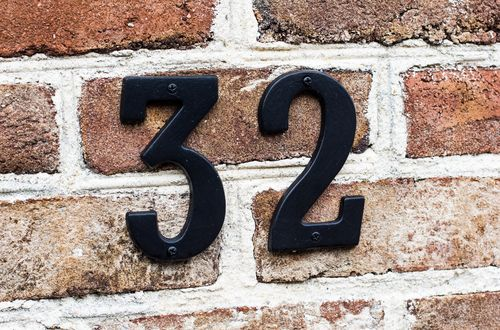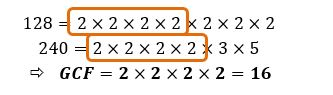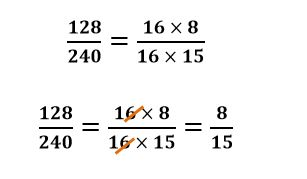# How to cancel fractions by determining the GCF

Want to find out how to cancel fractions by determining the GCF? We've provided a step-by-step guide of how to cancel fractions by determining the GCF to teach you everything you need to know.## Step 1: Determine the greatest common factor (GCF)

Determine the greatest common factor (GCF) shared by the numerator and the denominator of the given fraction. So, to cancel factors in 96/144 to simplify it, we first look for the GCF of 96 and 144.

## Step 2: Rewrite as products of the GCF

Rewrite the numerator and denominator of the fraction as products of the GCF. So, we need to express 96 and 144 as product of 48.

## Step 3: Simplify the fraction

Cancel out the GCF to simplify the fraction.

## Examples of how to cancel fractions by determining GCF

Q1) Cancel out the GCF to find the lowest term of 128/240

First, let’s determine the greatest common factor of 128 and 240.Express 128 and 240 as products of the GCF. Cancel out the GCF to simplify the fraction.This means that 128/240 is equal to 8/15 when simplified to its lowest term.

#### Join today

The fastest way to practice

Unlock our complete testing platform and improve faster that ever.